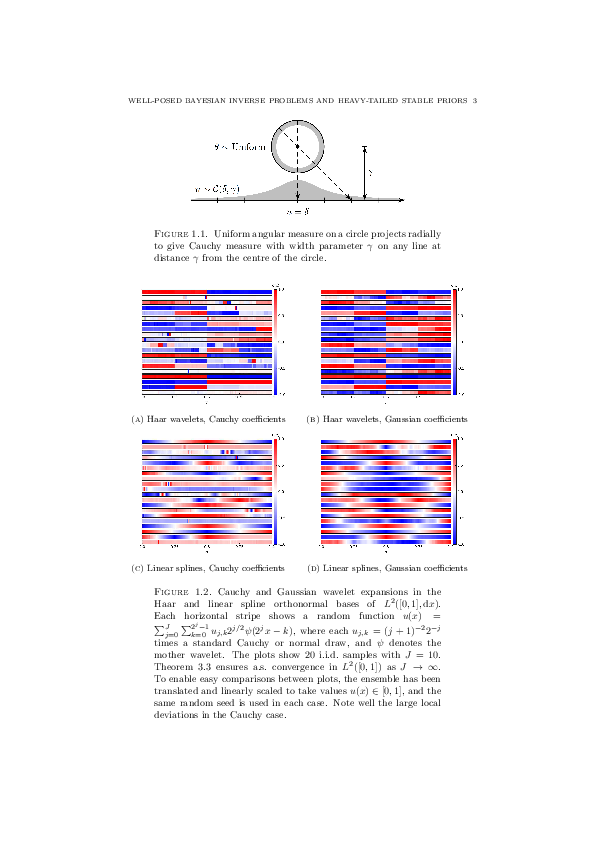# Tim Sullivan

### #cauchy-distribution

Clear Search### Dilations of Cauchy measures in Electron. Commun. Prob.

“Quasi-invariance of countable products of Cauchy measures under non-unitary dilations”, by Han Cheng Lie and myself, has just appeared online in Electronic Communications in Probability. This main result of this article can be understood as an analogue of the celebrated Cameron–Martin theorem, which characterises the directions in which an infinite-dimensional Gaussian measure can be translated while preserving equivalence of the original and translated measure; our result is a similar characterisation of equivalence of measures, but for infinite-dimensional Cauchy measures under dilations instead of translations.

H. C. Lie and T. J. Sullivan. “Quasi-invariance of countable products of Cauchy measures under non-unitary dilations.” Electronic Communications in Probability 23(8):1–6, 2018. doi:10.1214/18-ECP113

Abstract. Consider an infinite sequence $$(U_{n})_{n \in \mathbb{N}}$$ of independent Cauchy random variables, defined by a sequence $$(\delta_{n})_{n \in \mathbb{N}}$$ of location parameters and a sequence $$(\gamma_{n})_{n \in \mathbb{N}}$$ of scale parameters. Let $$(W_{n})_{n \in \mathbb{N}}$$ be another infinite sequence of independent Cauchy random variables defined by the same sequence of location parameters and the sequence $$(\sigma_{n} \gamma_{n})_{n \in \mathbb{N}}$$ of scale parameters, with $$\sigma_{n} \neq 0$$ for all $$n \in \mathbb{N}$$. Using a result of Kakutani on equivalence of countably infinite product measures, we show that the laws of $$(U_{n})_{n \in \mathbb{N}}$$ and $$(W_{n})_{n \in \mathbb{N}}$$ are equivalent if and only if the sequence $$(| \sigma_{n}| - 1 )_{n \in \mathbb{N}}$$ is square-summable.

Published on Wednesday 21 February 2018 at 09:30 UTC #publication #electron-commun-prob #cauchy-distribution #lie### Heavy-tailed stable priors in Inverse Problems and Imaging

The final version of “Well-posed Bayesian inverse problems and heavy-tailed stable quasi-Banach space priors” has now been published online in Inverse Problems and Imaging; the print version will be available in October.

T. J. Sullivan. “Well-posed Bayesian inverse problems and heavy-tailed stable quasi-Banach space priors.” Inverse Problems and Imaging 11(5):857–874, 2017. doi:10.3934/ipi.2017040

Published on Wednesday 19 July 2017 at 15:30 UTC #publication #bayesian #inverse-problems #cauchy-distribution #inverse-problems-and-imaging### Preprint: Bayesian inversion with heavy-tailed stable priors

A revised version of “Well-posed Bayesian inverse problems and heavy-tailed stable quasi-Banach space priors” has been released on arXiv today. Among other improvements, the revised version incorporates additional remarks on the connection to the existing literature on stable distributions in Banach spaces, and generalises the results of the previous version of the paper to quasi-Banach spaces, which are like complete normed vector spaces in every respect except that the triangle inequality only holds in the weakened form

$$\| x + y \| \leq C ( \| x \| + \| y \| )$$

for some constant $$C \geq 1$$.

Published on Monday 21 November 2016 at 11:30 UTC #publication #preprint #bayesian #inverse-problems #cauchy-distribution